# IIT JEE Physics Video Lectures

Every year students in mass number prepare for the IIT JEE exams. The main focus of the students is to gather all the knowledge they can through online mediums as it is easy to access. IIT JEE, Physics is the most difficult section to prepare amongst the students. The aspirants every year look out for the latest information and changes in the syllabus.

CLEAREXAM provides the Best Physics Video Lectures for IIT JEE which the students can refer to prepare for their exams. These video lectures, can be accessed by every student at the ease of their mobile phones and computers. Without paying the high cost of the coaching centers, students now have access to the IIT JEE physics video lectures, imparted by the best faculty. The video lectures curated by the best of the IIT faculties have been initially aggregated and then segregated by our team at CLEAREXAM.

According to the syllabus, Mechanics have the highest weightage of 35%, Current Electricity and EMI has 20% weightage, Optics and Modern Physics has 17%, Electrostatics and Magnetism has 17% and Heat & Thermodynamics has 9% weightage of the questions which come in the exam.

The IIT JEE physics video lectures are the best in class video coachings for each section of the syllabus. Aspirants can refer to the video lectures provided by the IITians for free, to rev up there knowledge of the topics in a short span of time. Our initiative is to save the time of the students to search for relevant video lectures required to study the topics. So the next time you want to stop for the IIT JEE physics video lectures, head towards CLEAREXAM.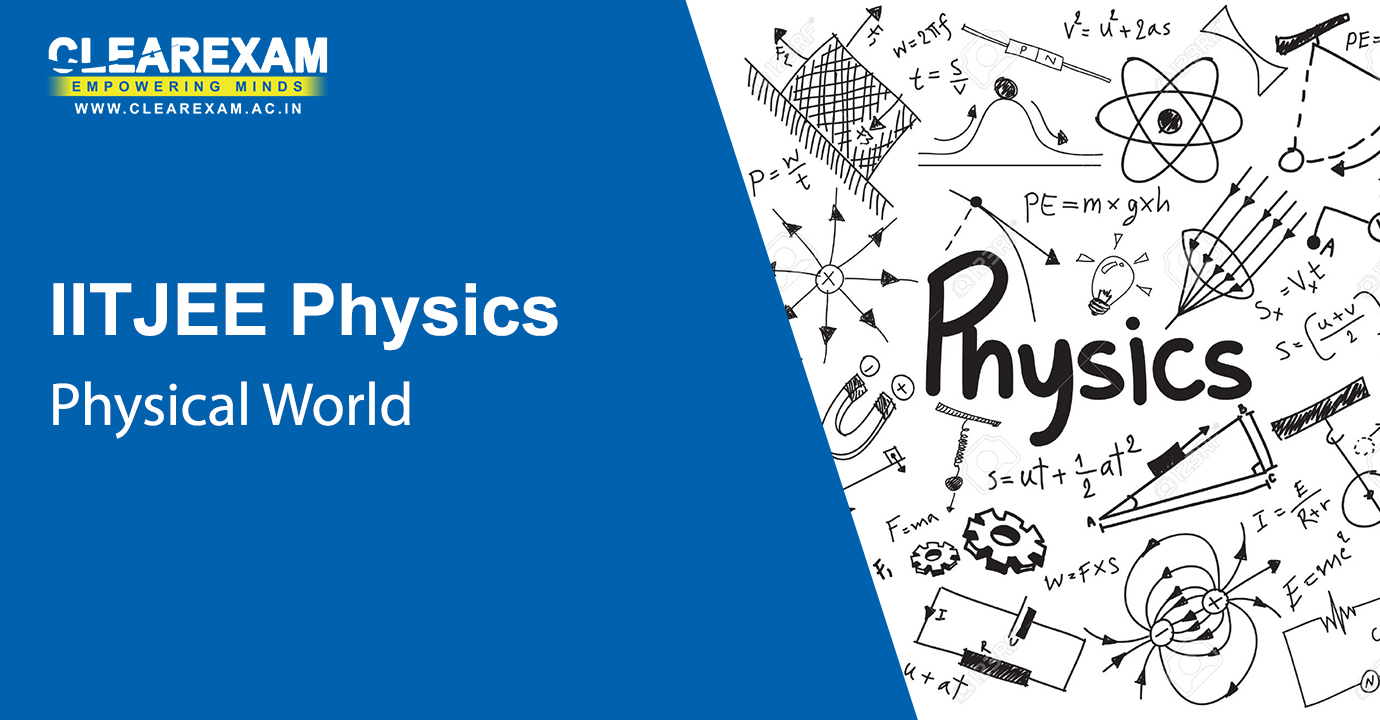Physical World

The first section of the syllabus has IIT JEE Physics Physical World Video Lectures which refers to the topics such as Definition of physics, Excitement of physics, fundamental forces in nature, physics, technology & society and nature of physical laws. These video lectures cover the topics effectively and through these, the students can grasp the information more quickly which has been taught. Refer to our video lectures to get the proper insights of this chapter.

Physical World
Chapter - Physical World
Subject - IIT JEE Physics
Excitement in Physics
Chapter - Physical World
Subject - IIT JEE PhysicsUnits and Measurements

The topics covered in the IIT JEE Physics Units and Measurements Video Lectures are the international system of units, measurement of length, mass & time, precision of instruments & errors in measurement, significant figures, dimensions of physical quantities, dimensional formulae & dimensional equations, dimensional analysis & its applications. There are 4 videos which explain the topic well and in the best possible way.

Dimensions of Physical Quantities, Dimensional Analysis and its Applications
Chapter - Units and Measurements
Subject - IIT JEE Physics
Introduction to Error Analysis
Chapter - Units and Measurements
Subject - IIT JEE Physics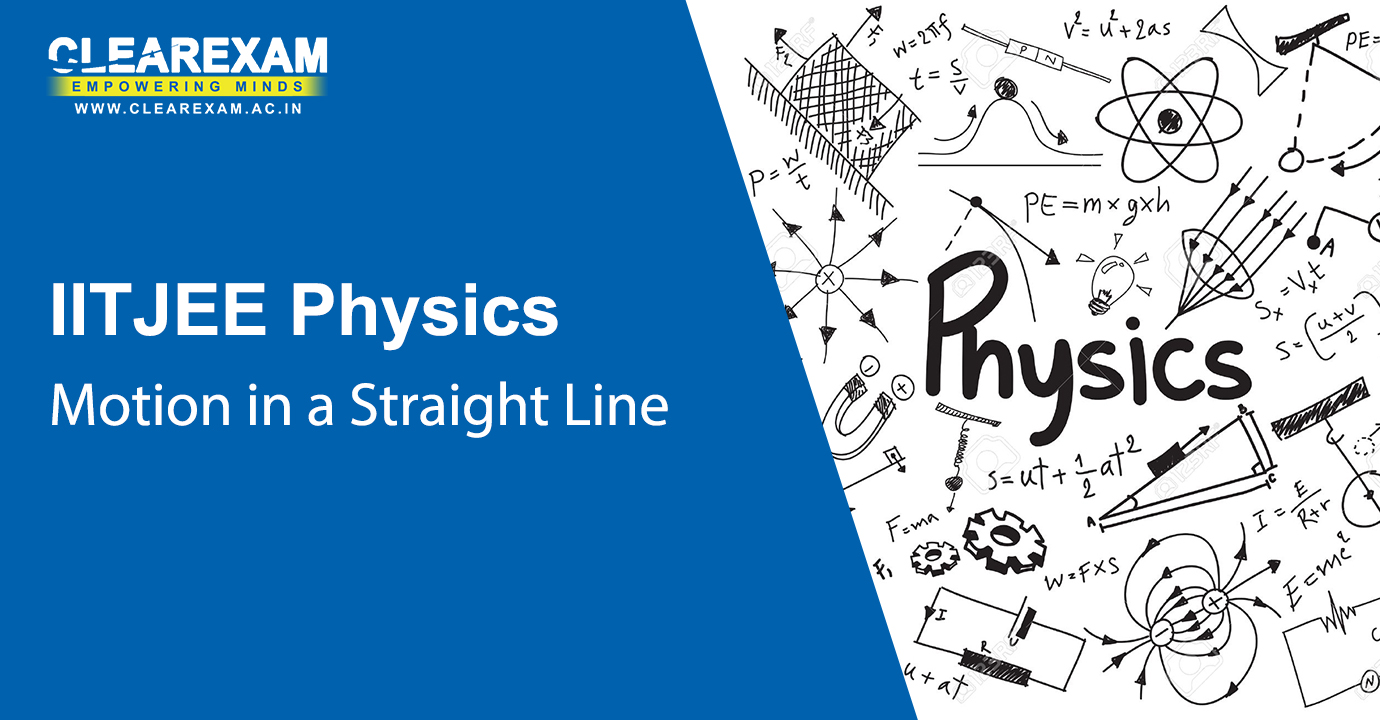Motion in a Straight Line

Complete this topic in an interactive way by studying through our video lectures. IIT JEE Physics Motion in a Straight Line Video Lectures has topics covered such as position, path length & displacement, average velocity & average speed, instantaneous velocity & speed, acceleration, kinematic equations for uniformly accelerated motion and relative velocity. All the lectures in this section are of 1 hour each which explain the topics well.

Introduction to Kinematics: Basic Mathematical Concepts
Chapter - Motion in a Straight Line
Subject - IIT JEE Physics
Relative Velocity and Examples
Chapter - Motion in a Straight Line
Subject - IIT JEE PhysicsMotion in a Plane

The IIT JEE Physics Motion in a Plane Video Lectures refers to topics like scalars & vectors, multiplication of vectors by real numbers, addition & subtraction of vectors in graphical method, resolution of vectors, vector addition in analytical method, motion in a plane, motion in a plane with constant acceleration, relative velocity in two dimensions, projectile motion and uniform circular motion. The best possible faculty has explained all the topics for the students to understand in a short span of time.

Introduction to Vectors
Chapter - Motion in a Plane
Subject - IIT JEE Physics
Introduction to Vector Operations
Chapter - Motion in a Plane
Subject - IIT JEE Physics
Planer Motion
Chapter - Motion in a Plane
Subject - IIT JEE Physics
Problems in Kinematics
Chapter - Motion in a Plane
Subject - IIT JEE PhysicsLaws of Motion

Refer to our video lectures to understand the topics in detail. In this section there are IIT JEE Physics Laws of Motion Video Lectures whichcovers topics such as Aristotle’s Fallacy, the law of inertia, Newton's first law of motion, Newton’s second law of motion, Newton’s third law of motion, conservation of momentum, equilibrium of a particle, common forces in mechanics, circular motion and solving problems in mechanics.

Newton's First Law of Motion
Chapter - Laws of Motion
Subject - IIT JEE Physics
Newton's Second and Third Laws of Motion
Chapter - Laws of Motion
Subject - IIT JEE Physics
Problem Solving Newton's Second Law of Motion
Chapter - Laws of Motion
Subject - IIT JEE Physics
Forces on Bodies: Contact and Non-Contact Forces
Chapter - Laws of Motion
Subject - IIT JEE Physics
Forces on Bodies: Systems involving Strings or Springs
Chapter - Laws of Motion
Subject - IIT JEE Physics
Forces on Bodies: Procedure to Solve Problems
Chapter - Laws of Motion
Subject - IIT JEE Physics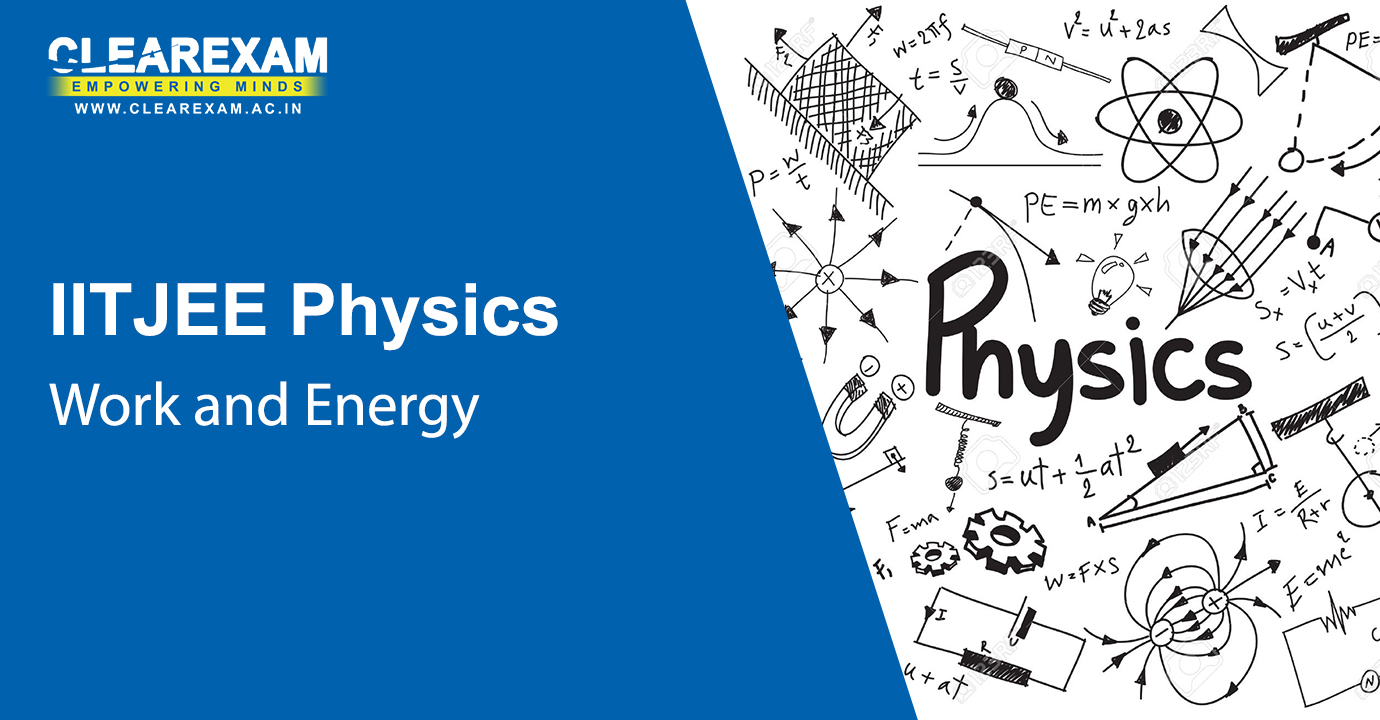Work and Energy

Learn and grasp the topics in this chapter through our video lectures. IIT JEE Physics Work and Energy Video Lectures has topics covered such as notions of work and kinetic energy, work, kinetic energy, work done by a variable force, work-energy theorem for a variable force, concept of potential energy, conservation of mechanical energy, potential energy of a spring, the law of conservation of energy, power and collisions.

Constant and Variable Forces, Kinetic Energy
Chapter - Work and Energy
Subject - IIT JEE Physics
Work energy and Impulse Momentum Principles, Conservation of Momentum
Chapter - Work and Energy
Subject - IIT JEE Physics
Impact and Collision
Chapter - Work and Energy
Subject - IIT JEE Physics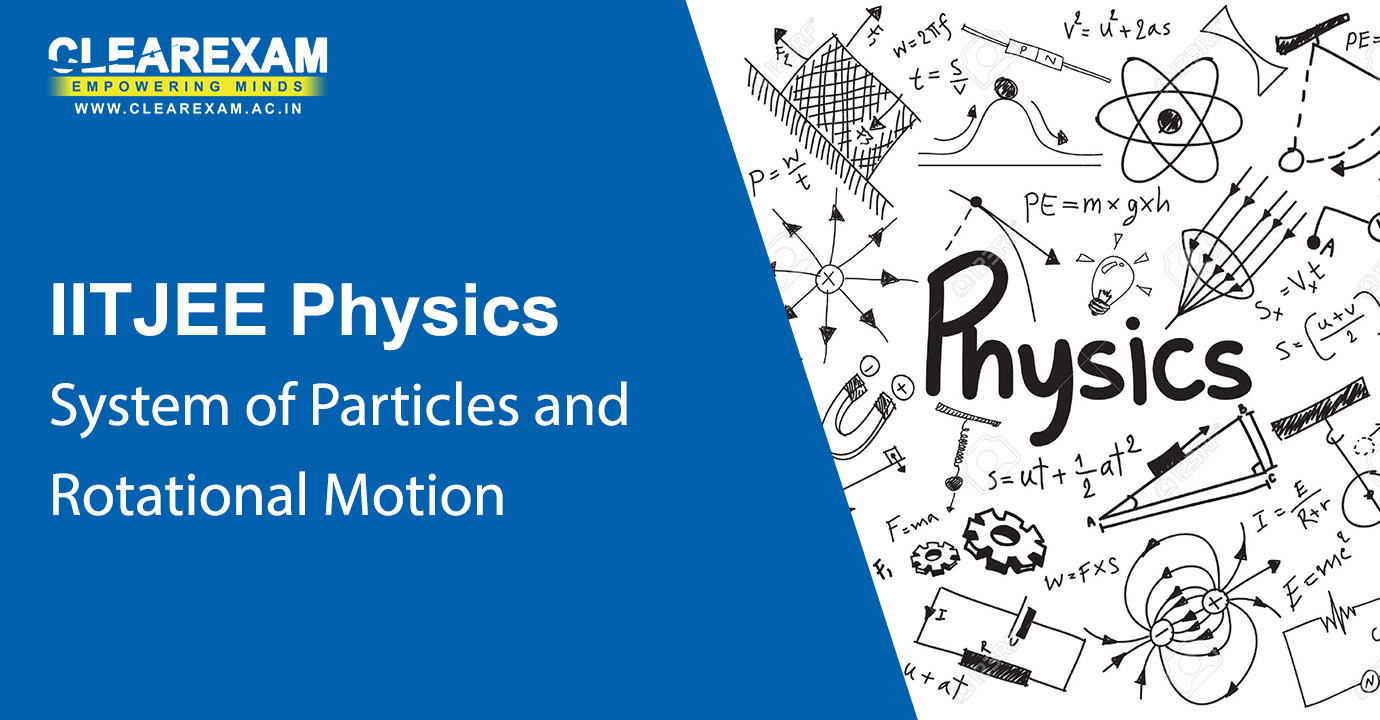System of Particles and Rotational Motion

The IIT JEE Physics System of Particles and Rotational Motion Video Lectures consists of topics such as centre of mass, motion of centre of mass, linear momentum of a system of particles, vector product of two vectors, angular velocity & its relation with linear velocity, torque & angular momentum, equilibrium of a rigid body, moment of inertia, theorems of perpendicular & parallel axes, kinematics of rotational motion about a fixed axis, dynamics of rotational motion about a fixed axis, angular momentum in case of rotation about a fixed axis and rolling motion. The video lectures under this section have proved to be very effective among students to understand the concepts.

Introduction, Center of Mass
Chapter - System of Particles and Rotational Motion
Subject - IIT JEE Physics
Motion of Center of Mass, Relative Motion and Reduced Mass
Chapter - System of Particles and Rotational Motion
Subject - IIT JEE Physics
Vector Products, Angular Velocity and Angular Acceleration
Chapter - System of Particles and Rotational Motion
Subject - IIT JEE Physics
Torque and Angular Momentum
Chapter - System of Particles and Rotational Motion
Subject - IIT JEE Physics
Equilibrium of a Rigid Body, Moments and Center of Gravity
Chapter - System of Particles and Rotational Motion
Subject - IIT JEE Physics
Moment of Inertia, Theorems of Perpendicular and Parallel Axes
Chapter - System of Particles and Rotational Motion
Subject - IIT JEE Physics
Rotational motion about a Fixed Axis - Kinematics and Dynamics
Chapter - System of Particles and Rotational Motion
Subject - IIT JEE Physics
Rotational motion about a Fixed Axis - Angular Momentum
Chapter - System of Particles and Rotational Motion
Subject - IIT JEE Physics
Problem Session-1: Motion of System of Particles and Rigid Bodies
Chapter - System of Particles and Rotational Motion
Subject - IIT JEE Physics
Problem Session-2: Motion of System of Particles and Rigid Bodies
Chapter - System of Particles and Rotational Motion
Subject - IIT JEE PhysicsGravitation

Our video lectures designed by the best faculty, who make the topics easy to understand.The topics in IIT JEE Physics Gravitation Video Lectures are kepler’s laws, universal law of gravitation, the gravitational constant, acceleration due to gravity of the earth, acceleration due to gravity below & above the surface of earth, gravitational potential energy, escape speed, earth satellites, energy of an orbiting satellite, geostationary & polar satellites and weightlessness.

Introduction to Gravitation
Chapter - Gravitation
Subject - IIT JEE Physics
Conservation Laws, Fundamental Forces, Estimation of Distances
Chapter - Gravitation
Subject - IIT JEE Physics
More on Distances, Galilean Law, Kepler Laws, Centripetal Forces
Chapter - Gravitation
Subject - IIT JEE Physics
Galilean Law, Kepler Laws, Centripetal Forces, Gravitational Law
Chapter - Gravitation
Subject - IIT JEE Physics
Determination of Gravitational Constant (G)
Chapter - Gravitation
Subject - IIT JEE Physics
Tidal Forces, Gravitational Potential Energy, Energy Conservation
Chapter - Gravitation
Subject - IIT JEE Physics
Gravitational Potential and Potential Energy
Chapter - Gravitation
Subject - IIT JEE Physics
Escape Velocity, Natural and Artificial Satellites
Chapter - Gravitation
Subject - IIT JEE Physics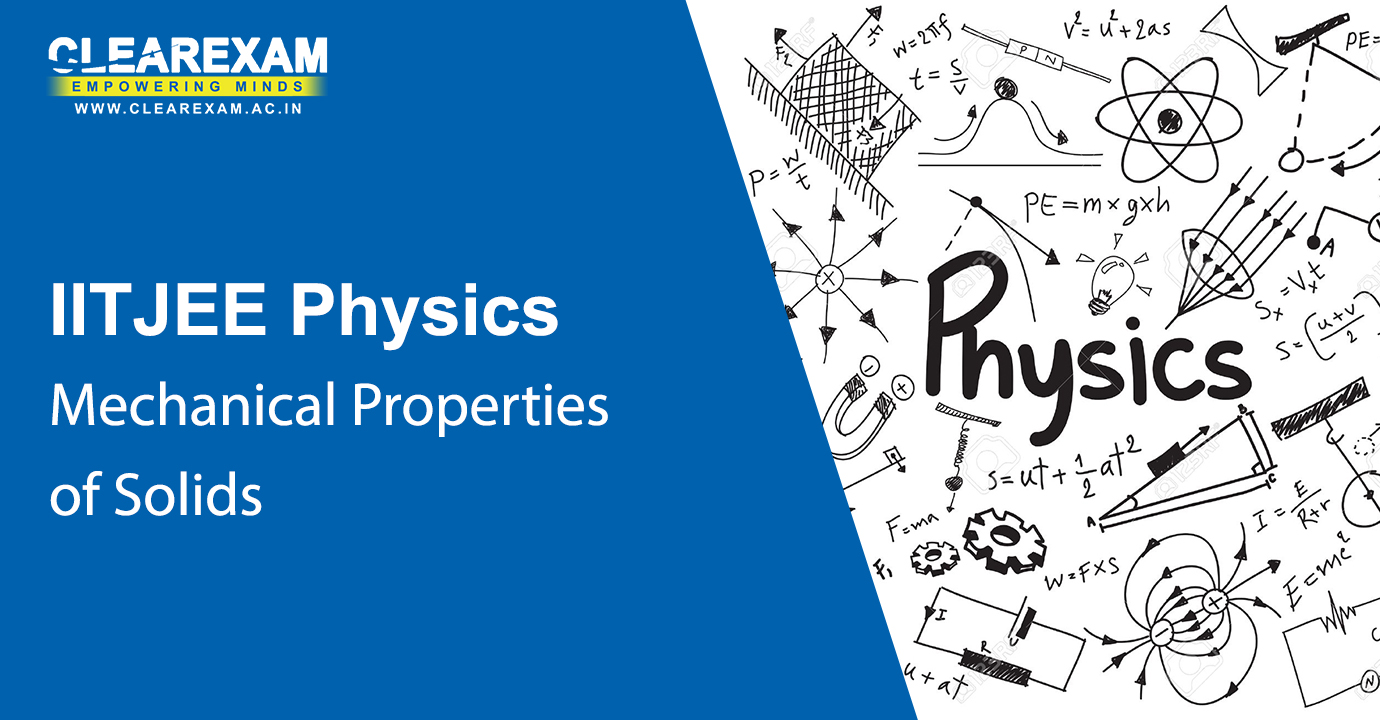Mechanical Properties of Solids

The IIT JEE Physics Mechanical Properties of Solids Video Lectures has topics such as elastic behaviour of solids, stress & strain, Hooke’s law, stress-strain curve, elastic moduli and applications of elastic behaviour of materials. These are 1 hour each video lectures which the students can refer to, in order to perceive the chapters in a better way.

Elastic Behaviour of Solids, Modulus of Elasticity, Stress-Strain, Hooke's Law
Chapter - Mechanical Properties of Solids
Subject - IIT JEE Physics
Experimental Determination of Young's Modulus
Chapter - Mechanical Properties of Solids
Subject - IIT JEE Physics
Elastic Properties of Human Body
Chapter - Mechanical Properties of Solids
Subject - IIT JEE PhysicsMechanical Properties of Fluids

IIT JEE Physics Mechanical Properties of Fluids Video Lectures has topics such as Pressure, Streamline Flow, Bernoulli’s Principle, Viscosity, Reynolds Number and Surface Tension. This topic talks about the common physical properties of liquids & gases. Refer to our video lectures to get the in depth knowledge of the topic like never before.

Density, Pressure due to Static Fluids
Chapter - Mechanical Properties of Fluids
Subject - IIT JEE Physics
Atmospheric Pressure and Gauge Pressure, Measurement of Pressure
Chapter - Mechanical Properties of Fluids
Subject - IIT JEE Physics
Pascal's Law, Buoyancy and Archimedes Principle, Streamline Flow, Equation of Continuity
Chapter - Mechanical Properties of Fluids
Subject - IIT JEE Physics
Bernoulli's Principle, Venturimeter, Surface Tension
Chapter - Mechanical Properties of Fluids
Subject - IIT JEE Physics
Viscosity, Poiseuille's Law
Chapter - Mechanical Properties of Fluids
Subject - IIT JEE PhysicsKinetic Theory of Gases

In this section there is IIT JEE Physics Kinetic Theory of Gases Video Lectures which has topics such as molecular nature of matter, behaviour of gases, kinetic theory of an ideal gas, law of equipartition of energy, specific heat capacity and mean free path. The video lectures explain the concepts clearly for the students and the duration of each lecture is of 1 hour. We provide the best video lectures which help the students to grasp the information much more easily.

Microscopic and Macroscopic Apporach to Thermal Properties
Chapter - Kinetic Theory of Gases
Subject - IIT JEE Physics
Kinetic Theory of Gases & Equation of Ideal Gas
Chapter - Kinetic Theory of Gases
Subject - IIT JEE Physics
Equipartition of Energy
Chapter - Kinetic Theory of Gases
Subject - IIT JEE Physics
Mean Freepath and Non-Ideal Gas
Chapter - Kinetic Theory of Gases
Subject - IIT JEE Physics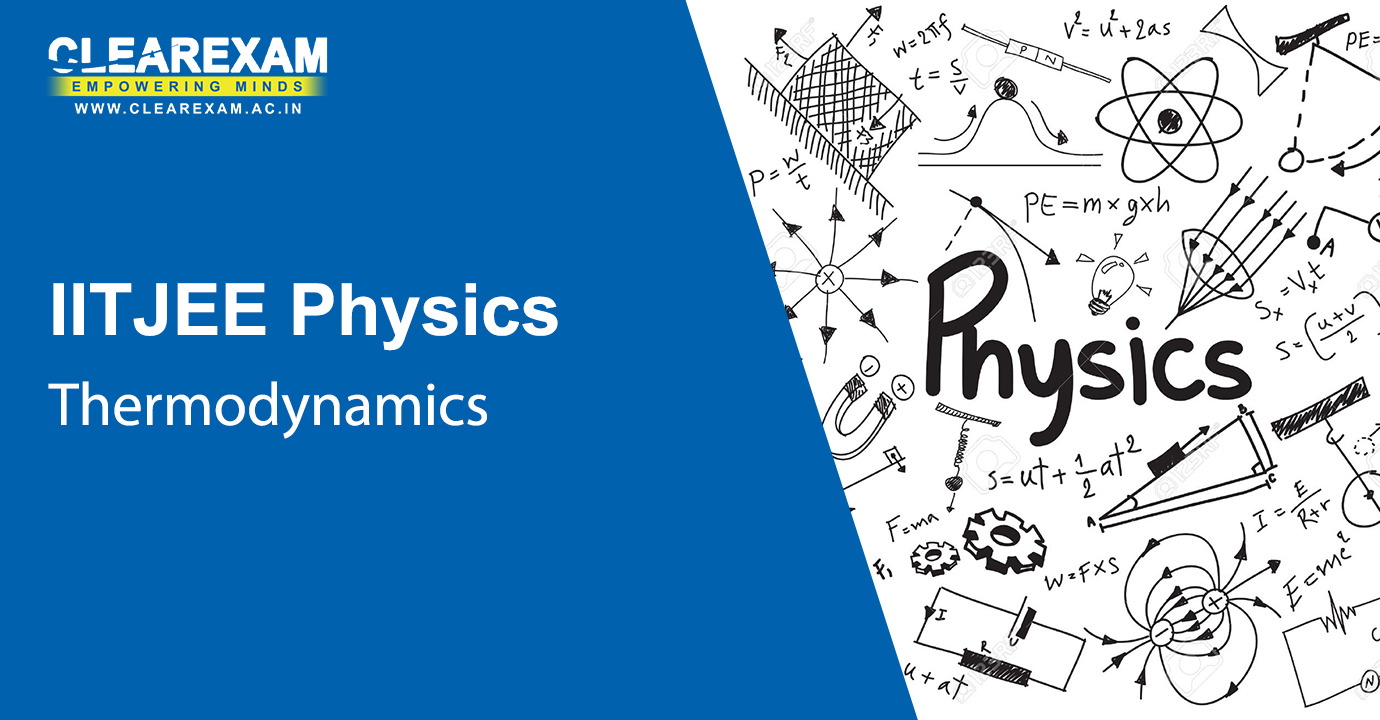Thermodynamics

Under the topic of Thermodynamics, there are insightful information provided of the subject for the students to perceive the concepts better. The topics in explained in IIT JEE Physics Thermodynamics Video Lectures are like thermal equilibrium, the zeroth law of thermodynamics, heat, internal energy & work, first law of thermodynamics, specific heat capacity, thermodynamic state variables & equation of state, thermodynamic processes, heat engines, refrigerators & heat pumps, second law of thermodynamics, reversible & irreversible processes and carnot engine.

Introduction to Thermodynamics - First Law and Internal Energy
Chapter - Thermodynamics
Subject - IIT JEE Physics
First Law: Work Done in Different Thermodynamics Processes
Chapter - Thermodynamics
Subject - IIT JEE Physics
Heat Engine and Refrigerators
Chapter - Thermodynamics
Subject - IIT JEE Physics
Carnot Engine and Carnot Theorem
Chapter - Thermodynamics
Subject - IIT JEE Physics
Entropy and T-S Diagram
Chapter - Thermodynamics
Subject - IIT JEE Physics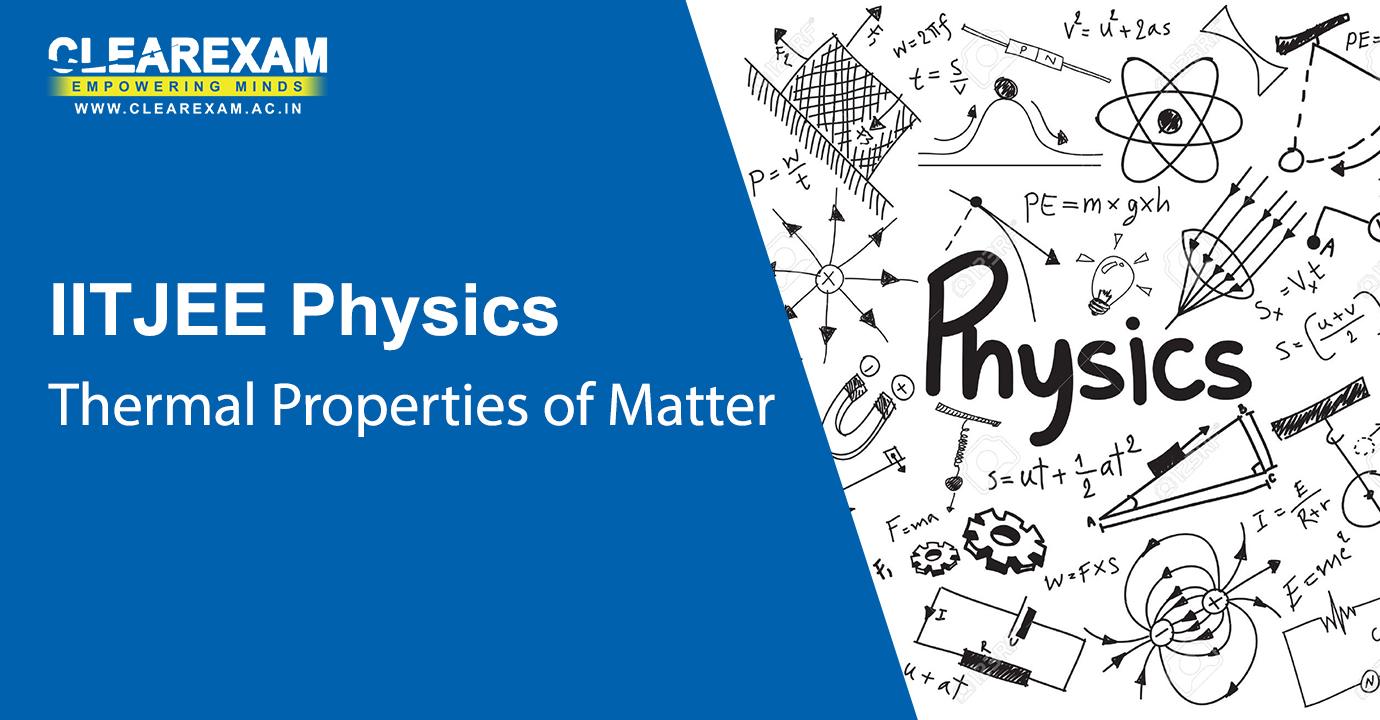Thermal Properties of Matter

The IIT JEE Physics Thermal Properties of Matter Video Lectures refers to the topics such as temperature & heat, measurement of temperature, ideal-gas equation & absolute temperature, thermal expansion, specific heat capacity, calorimetry, change of state, heat transfer and Newton’s law of cooling. Refer to our video lectures to get the best knowledge on the Thermal Properties of Matter.

Concept of Temperature, Thermal Expansion
Chapter - Thermal Properties of Matter
Subject - IIT JEE Physics
Specific Heat Capacity, Calorimetry, Change of State
Chapter - Thermal Properties of Matter
Subject - IIT JEE Physics
Heat Transfer, Newton's Law of Cooling
Chapter - Thermal Properties of Matter
Subject - IIT JEE Physics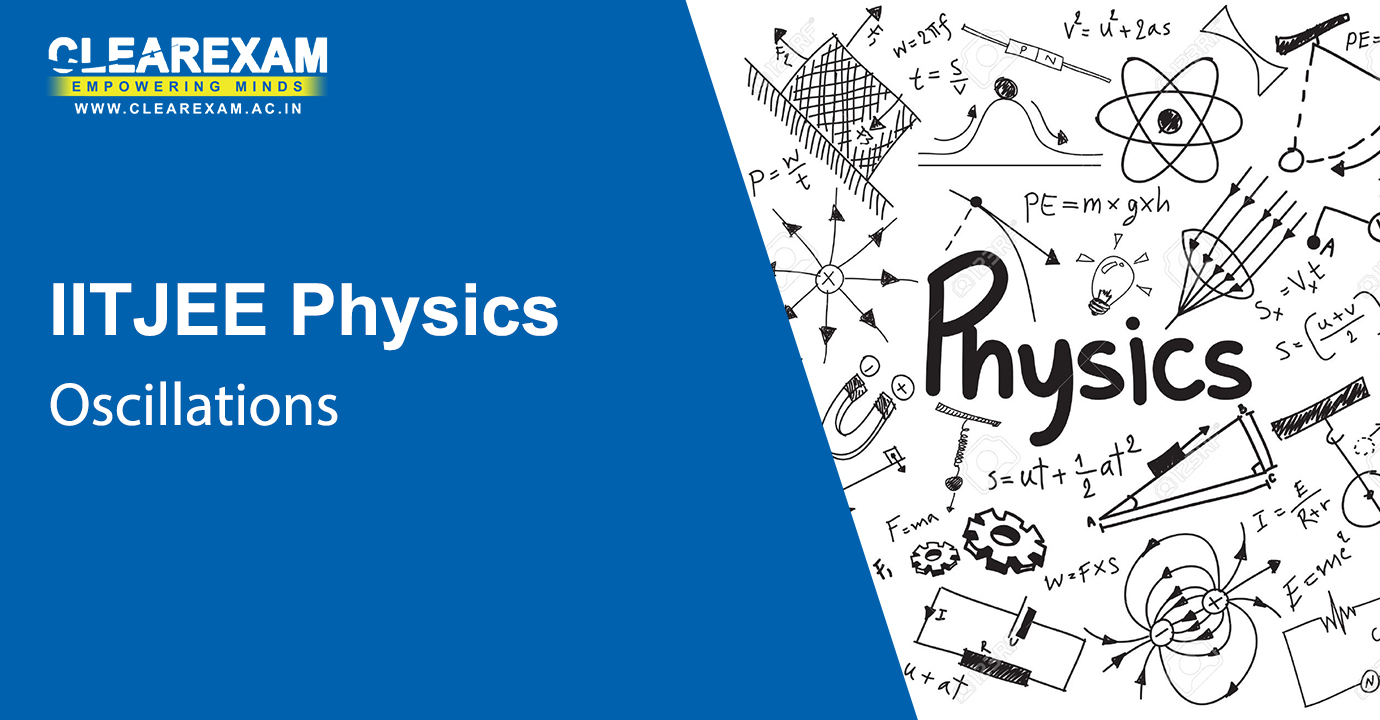Oscillations

Study the concepts of Oscillations better with these video lectures which have helped the students to grasp the concept better. IIT JEE Physics Oscillations Video Lectures has topics like periodic & oscillatory motions, simple harmonic motion, simple harmonic motion & uniform circular motion, velocity & acceleration in simple harmonic motion, force law for simple harmonic motion, energy in simple harmonic motion, some systems executing SHM, damped simple harmonic motion and forced oscillations & resonance.

Introduction to Periodic Motion
Chapter - Oscillations
Subject - IIT JEE Physics
Introduction to Simple Harmonic Motion
Chapter - Oscillations
Subject - IIT JEE Physics
Examples of Simple Harmonic Motion
Chapter - Oscillations
Subject - IIT JEE Physics
Damped Harmonic Oscillator
Chapter - Oscillations
Subject - IIT JEE PhysicsWaves

The video lectures explain about the concept of waves, how are they formed and communication which takes place through waves. This IIT JEE Physics Waves Video Lectures is talking about the transverse & longitudinal waves, displacement relation in a progressive wave, the speed of a travelling wave, the principle of superposition of waves, reflection of waves, beats and Doppler Effect.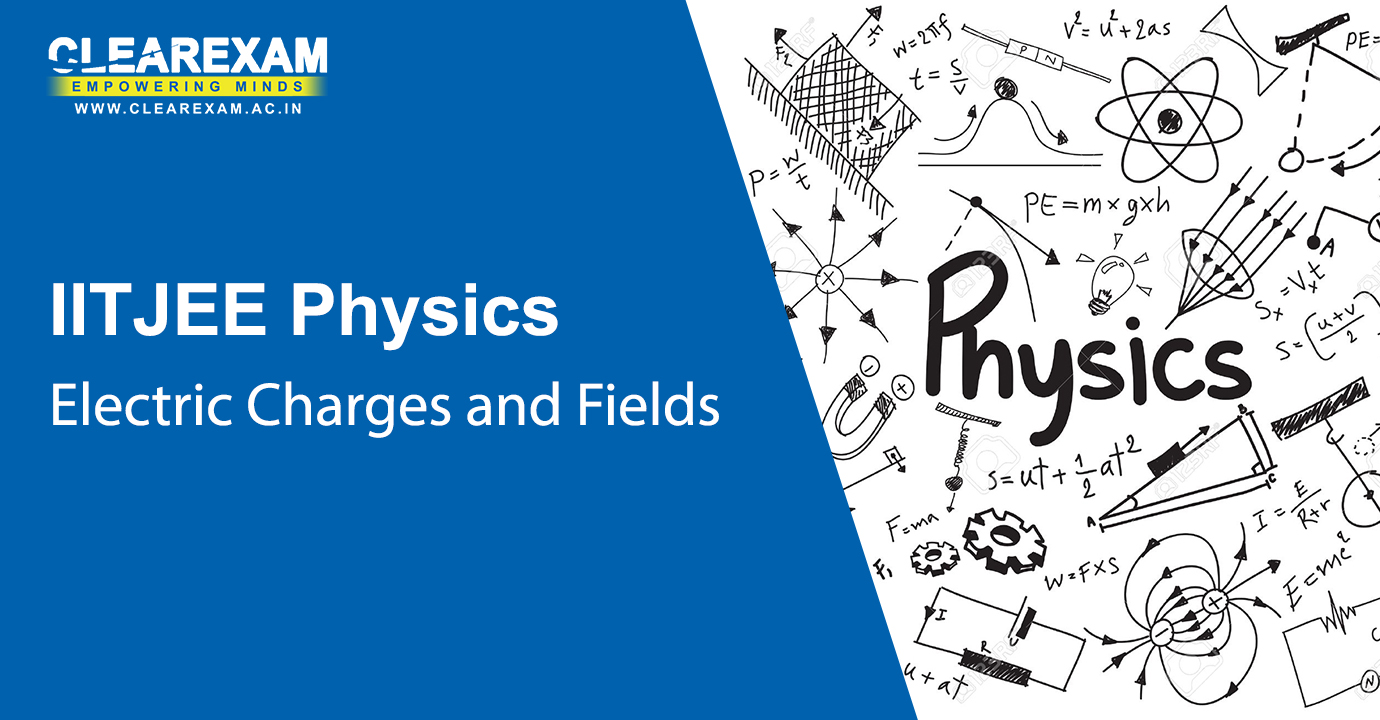Electric Charges and Fields

Study through our video lectures and make the best use of the concepts explained in an easy manner. The IIT JEE Physics Electric Charges and Fields Video Lectures contains topics such as electric charges, conductors & insulators, charging by induction, basic properties of electric charge, Coulomb's Law, forces between multiple charges, electric field, electric field lines, electric flux, electric dipole, dipole in a uniform external field, continuous charge distribution, Gauss’s Law, and Application of Gauss’s Law.

Concept of Charge and Coulomb's Law
Chapter - Electric Charges and Fields
Subject - IIT JEE Physics
Superposition Principle for Electrostatic Force
Chapter - Electric Charges and Fields
Subject - IIT JEE Physics
Concept of Electric Field, Electric Dipole
Chapter - Electric Charges and Fields
Subject - IIT JEE Physics
Field due to Dipole and Continuous Charge Distributions
Chapter - Electric Charges and Fields
Subject - IIT JEE Physics
Gauss's Law in Electrostatics
Chapter - Electric Charges and Fields
Subject - IIT JEE Physics
Applications of Gauss's Law
Chapter - Electric Charges and Fields
Subject - IIT JEE PhysicsElectrostatic Potential and Capacitance

In this section there are IIT JEE Physics Electrostatic Potential and Capacitance Video Lectures which cover topics such as Electrostatic Potential, potential due to a point charge, potential due to an electric dipole, potential due to a system of charges, equipotential surfaces, potential energy of a system of charges, potential energy in an external field, electrostatics of conductors, dielectrics & polarisation, capacitors & capacitance, the parallel plate capacitor, effect of dielectric on capacitance, combination of capacitors, energy stored in a capacitor and Van De Graaff Generator.

Electrostatic Potential and Potential Energy
Chapter - Electrostatic Potential and Capacitance
Subject - IIT JEE Physics
Potential due to Different Charge Distributions
Chapter - Electrostatic Potential and Capacitance
Subject - IIT JEE Physics
Electric field and Potential, Concept of Capacitance
Chapter - Electrostatic Potential and Capacitance
Subject - IIT JEE Physics
Cylindrical and Spherical Capacitors, Series and Parallel Combinations
Chapter - Electrostatic Potential and Capacitance
Subject - IIT JEE Physics
Energy stored in Capacitors, Field in Dielectrics, Gauss's Law in Dielectrics
Chapter - Electrostatic Potential and Capacitance
Subject - IIT JEE Physics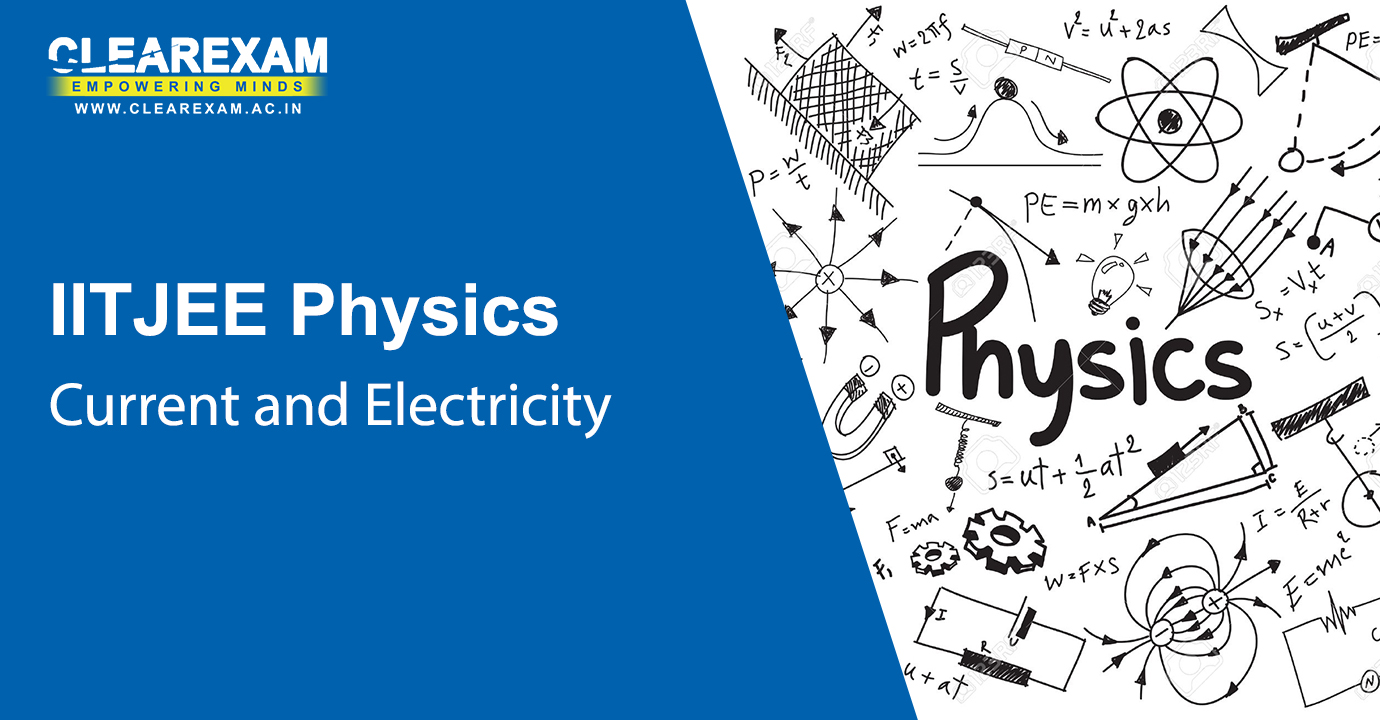Current Electricity

IIT JEE Physics Current Electricity Video Lectures contains the topics on Electric Current, Electric Currents in Conductors, Ohm’s Law, Drift of Electrons & the Origin of Resistivity, Limitations of Ohm’s Law, Resistivity of Various Materials, Temperature Dependence of Resistivity, Electrical Energy, Power, Combination of Resistors liked Series and Parallel, Cells, emf, Internal Resistance, Cells in Series and in Parallel, Kirchhoff’s Laws, Wheatstone Bridge, Meter Bridge and Potentiometer. We have provided the best faculty’s video lectures which will enhance the knowledge of the students.

Electric Current and Current Density
Chapter - Current Electricity
Subject - IIT JEE Physics
Drift Velocity and Resistance
Chapter - Current Electricity
Subject - IIT JEE Physics
Mobility and Temperature Dependence of Resistivity
Chapter - Current Electricity
Subject - IIT JEE Physics
Electromotive Force and Ohm's Law
Chapter - Current Electricity
Subject - IIT JEE Physics
Electrical Energy and Power
Chapter - Current Electricity
Subject - IIT JEE Physics
Series and Parallel Combination of Resistances
Chapter - Current Electricity
Subject - IIT JEE Physics
Equivalent Circuits
Chapter - Current Electricity
Subject - IIT JEE Physics
Series and Parallel Combinations of Cells
Chapter - Current Electricity
Subject - IIT JEE Physics
Kirchhoff's Laws
Chapter - Current Electricity
Subject - IIT JEE Physics
Wheatstone's Bridge, Meter Bridge and Potentiometer
Chapter - Current Electricity
Subject - IIT JEE Physics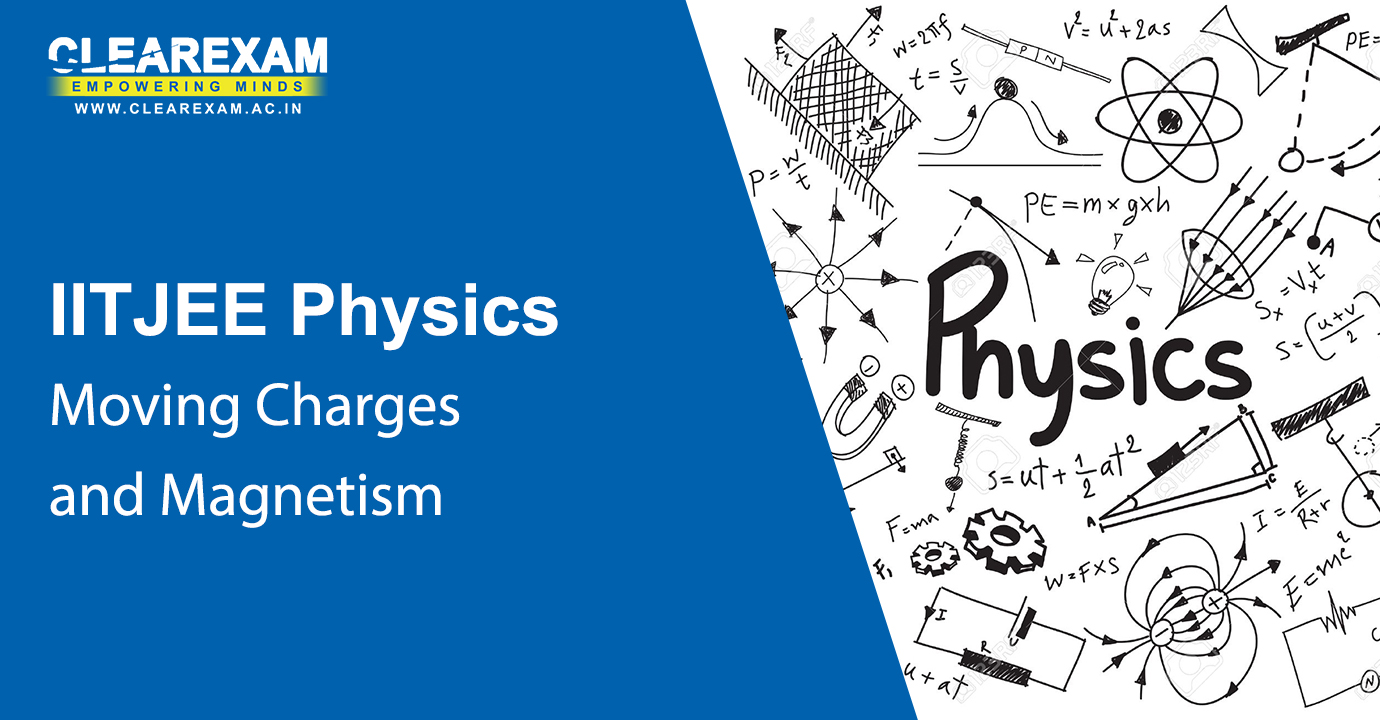Moving Charges and Magnetism

Refer to our video lectures which have the in depth knowledge within the given in 1 hour. While referring to IIT JEE Physics Moving Charges and Magnetism Video Lectures the following topics are addressed: Magnetic Force, Motion in a Magnetic Field, Motion in Combined Electric and Magnetic Fields, Magnetic Field due to a Current Element, Biot-Savart Law, Magnetic Field on the Axis of a Circular Current Loop, Ampere’s Circuital Law, the Solenoid & the Toroid, Force between Two Parallel Currents, the Ampere, Torque on a Current Loop, Magnetic Dipole and The Moving Coil Galvanometer.

Introduction to Magnetostatics, Biot Savart Law
Chapter - Moving Charges and Magnetism
Subject - IIT JEE Physics
Motion of Charges in the Presence of Electric and Magnetic Fields
Chapter - Moving Charges and Magnetism
Subject - IIT JEE Physics
Magnetic Field for a Straight Conductor and Ampere's Law
Chapter - Moving Charges and Magnetism
Subject - IIT JEE Physics
Generalization of Ampere's Law and its Applications
Chapter - Moving Charges and Magnetism
Subject - IIT JEE Physics
More Applications of Ampere's Law
Chapter - Moving Charges and Magnetism
Subject - IIT JEE Physics
Force and Torque due to Magnetic Field
Chapter - Moving Charges and Magnetism
Subject - IIT JEE Physics
Moving Coil Galvanometer, Magnetic Dipole
Chapter - Moving Charges and Magnetism
Subject - IIT JEE Physics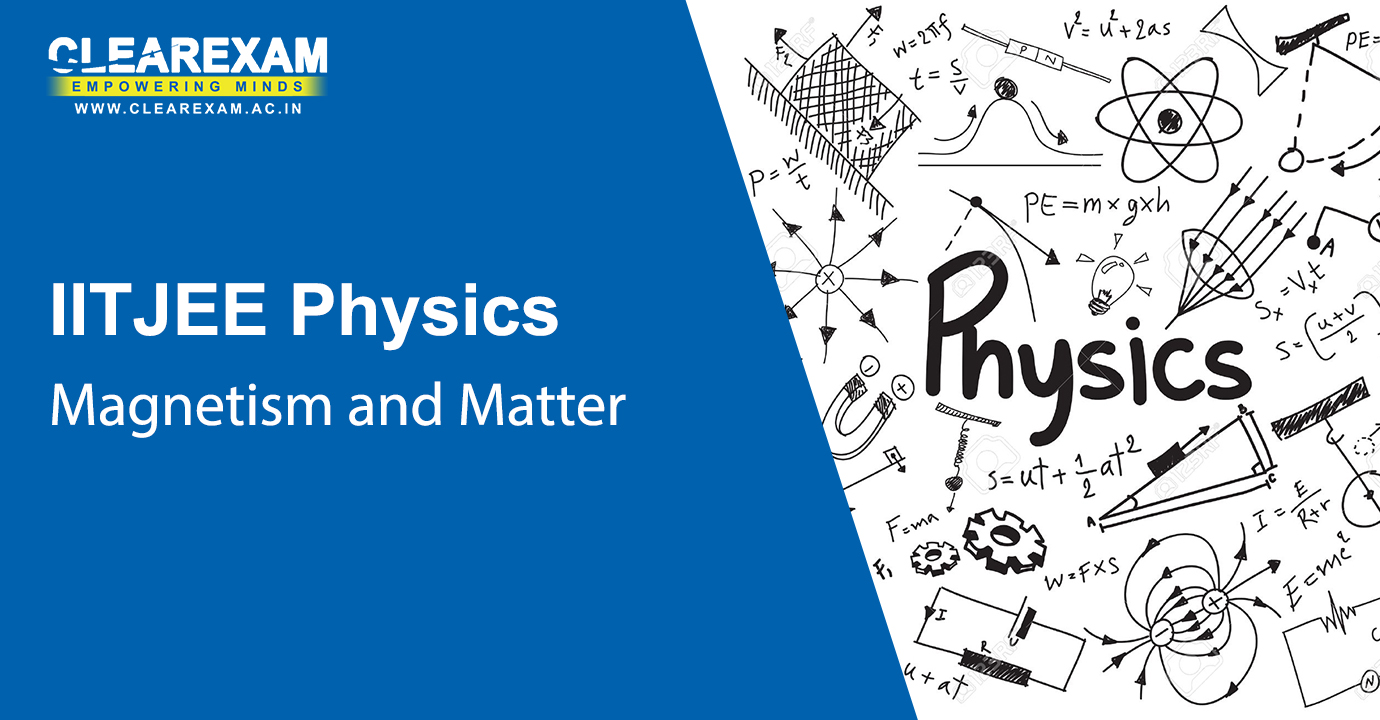Magnetism and Matter

This section has Magnetism and Matter explained in depth and each video has the best faculty teaching the topics in an effective way. The topics explained in IIT JEE Physics Magnetism and Matter Video Lectures are such as The Bar Magnet, Magnetism & Gauss’s Law, The Earth’s Magnetism, Magnetisation & Magnetic Intensity, Magnetic Properties of Materials and Permanent Magnets & Electromagnets.

Magnetic Dipole, Magnetization
Chapter - Magnetism and Matter
Subject - IIT JEE Physics
Magnetization and Application of Ampere's Law
Chapter - Magnetism and Matter
Subject - IIT JEE Physics
Diamagnetic, Paramagnetic and Ferromagnetic Materials, Magnetic field of the Earth
Chapter - Magnetism and Matter
Subject - IIT JEE Physics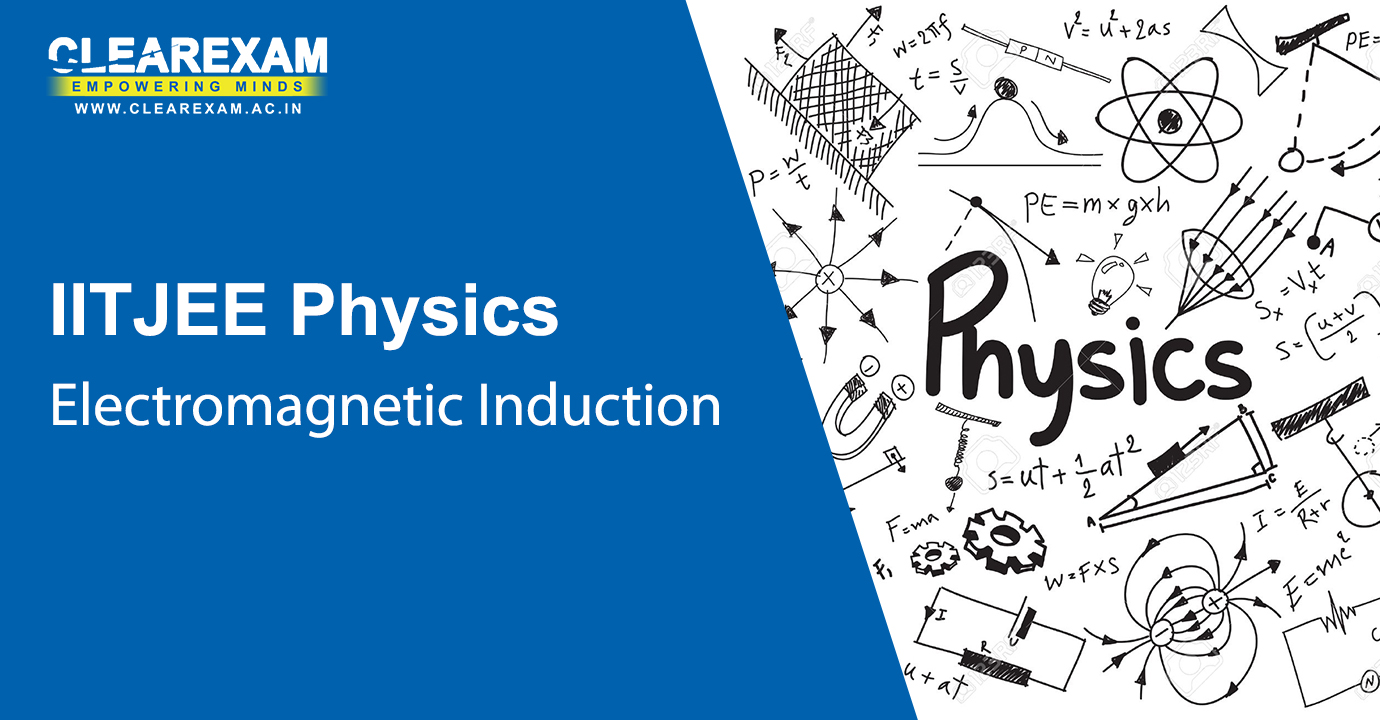Electromagnetic Induction

IIT JEE Physics Electromagnetic Induction Video Lectures has the following contents explained The Experiments of Faraday & Henry, Magnetic Flux, Faraday’s Law of Induction, Lenz’s Law & Conservation of Energy, Motional Electromotive Force, Energy Consideration A Quantitative Study, Eddy Currents, Inductance and AC Generator. Each video lecture under this topics has videos of 1 hour and they explain the concepts very easily. Refer to our video lectures to understand the concepts in an effective way.

Electromagnetic Induction
Chapter - Electromagnetic Induction
Subject - IIT JEE Physics
Faraday's Law of Induction, Induced EMF, Lenz's Law, Motional EMF
Chapter - Electromagnetic Induction
Subject - IIT JEE Physics
Eddy Currents, Inductance, Mutual and Self-Inductance
Chapter - Electromagnetic Induction
Subject - IIT JEE Physics
Energy in Magnetic Field
Chapter - Electromagnetic Induction
Subject - IIT JEE Physics
AC Generator
Chapter - Electromagnetic Induction
Subject - IIT JEE Physics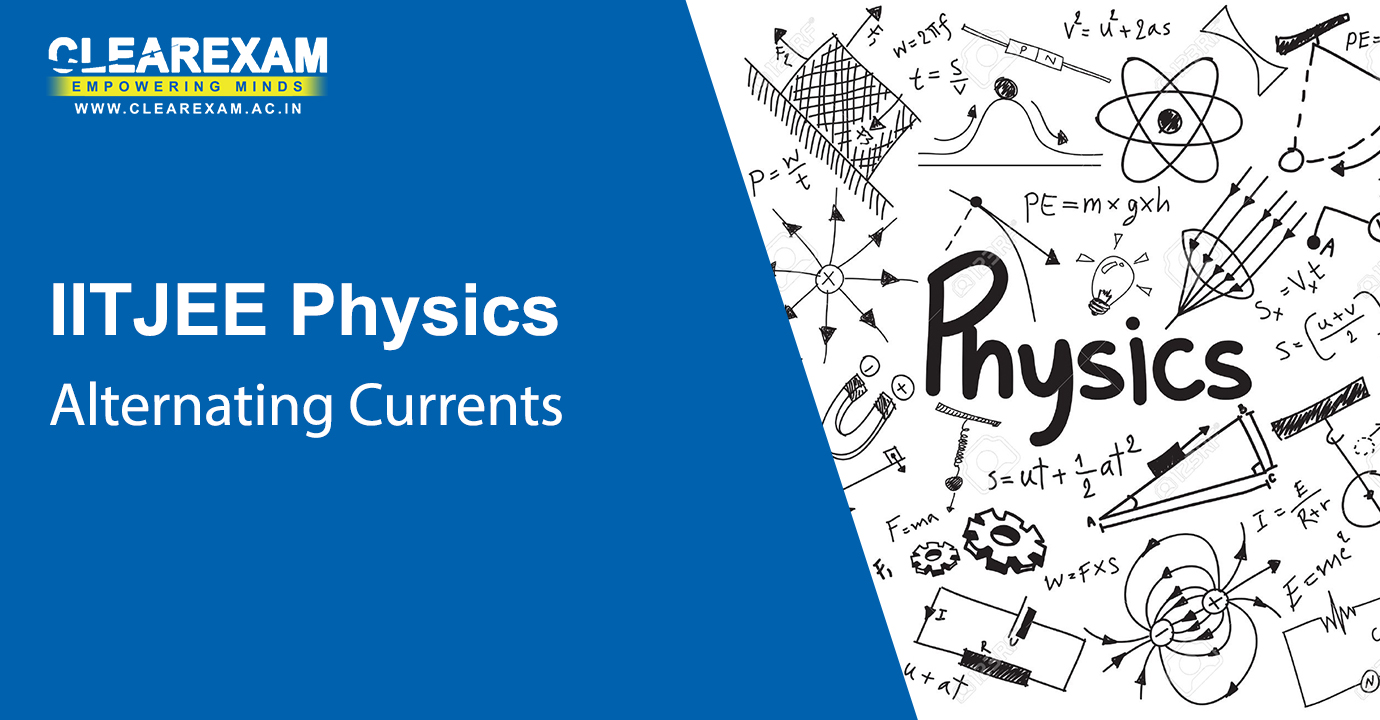Alternating Current

The IIT JEE Physics Alternating Current Video Lectures has the various topics covered such as AC Voltage Applied to a Resistor, Representation of AC Current & Voltage by Rotating Vectors-Phasors, AC Voltage Applied to an Inductor, AC Voltage Applied to a Capacitor, AC Voltage Applied to a Series LCR Circuit, Power in AC Circuit: The Power Factor, LC Oscillations and Transformers. Each video under this section has been specially curated for the students to perceive the concepts better.

Circuits with Resistance and Inductance
Chapter - Alternating Current
Subject - IIT JEE Physics
Capacitive Circuits
Chapter - Alternating Current
Subject - IIT JEE Physics
LCR Circuit: Graphical Solution
Chapter - Alternating Current
Subject - IIT JEE Physics
LCR Circuit: Analytical Solution, Resonance
Chapter - Alternating Current
Subject - IIT JEE Physics
LCR Circuit: Applications
Chapter - Alternating Current
Subject - IIT JEE Physics
LCR Circuit: Power Factor
Chapter - Alternating Current
Subject - IIT JEE Physics
LC Oscillations
Chapter - Alternating Current
Subject - IIT JEE Physics
Transformers
Chapter - Alternating Current
Subject - IIT JEE PhysicsElectromagnetic Waves

This section talks about the IIT JEE Physics Electromagnetic Waves Video Lectures which contains sections such as Displacement Current, Electromagnetic Waves and the Electromagnetic Spectrum. Each video under this section has lectures of 1 hour each which explain each and every concept in a comprehensible manner.

Concepts of Waves and Electromagnetic Waves
Chapter - Electromagnetic Waves
Subject - IIT JEE Physics
Maxwell's Equations and Electromagnetic Waves
Chapter - Electromagnetic Waves
Subject - IIT JEE Physics
Displacement Current
Chapter - Electromagnetic Waves
Subject - IIT JEE Physics
Problems in Electromagnetics: Electrostatics
Chapter - Electromagnetic Waves
Subject - IIT JEE Physics
Problems in Electromagnetics: Magnetic Fields, EM Waves
Chapter - Electromagnetic Waves
Subject - IIT JEE Physics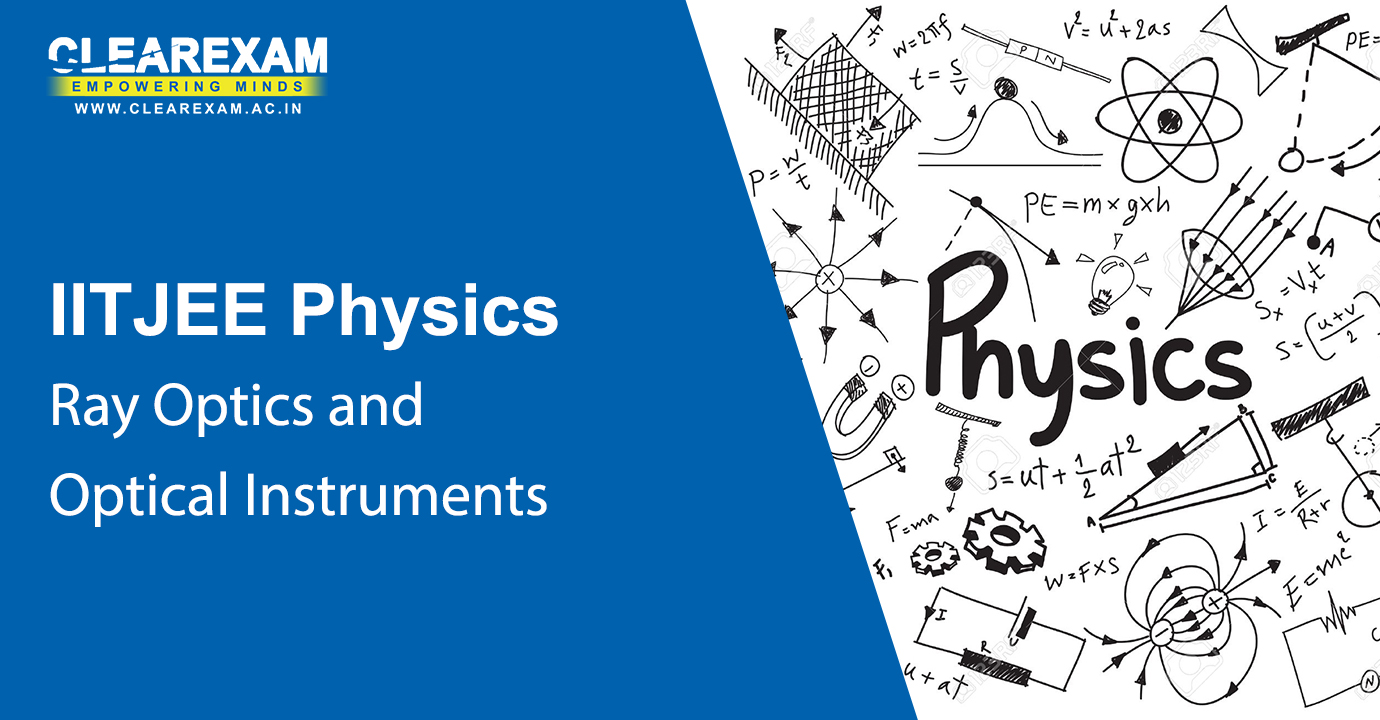Ray Optics and Optical Instruments

Brush up your concepts by viewing IIT JEE Physics Ray Optics and Optical Instruments Video Lectures which comprise of the following topics:Reflection of light by spherical mirrors, refraction, total internal reflection, refraction at spherical surfaces and by lenses, refraction through a prism, dispersion by a prism, some natural phenomena due to sun light and optical instruments.

What is Light-1
Chapter - Ray Optics and Optical Instruments
Subject - IIT JEE Physics
What is Light-2
Chapter - Ray Optics and Optical Instruments
Subject - IIT JEE Physics
General Introduction
Chapter - Ray Optics and Optical Instruments
Subject - IIT JEE Physics
Reflection of Light and Formation of Images
Chapter - Ray Optics and Optical Instruments
Subject - IIT JEE Physics
The Mirror Equation
Chapter - Ray Optics and Optical Instruments
Subject - IIT JEE Physics
Refraction of Light
Chapter - Ray Optics and Optical Instruments
Subject - IIT JEE Physics
Total Internal Reflection
Chapter - Ray Optics and Optical Instruments
Subject - IIT JEE Physics
Refraction at Spherical Surfaces and by Lenses
Chapter - Ray Optics and Optical Instruments
Subject - IIT JEE Physics
Power of a Lens and Combination of Thin Lenses in Contact
Chapter - Ray Optics and Optical Instruments
Subject - IIT JEE Physics
Refraction through a Prism and Dispersion
Chapter - Ray Optics and Optical Instruments
Subject - IIT JEE Physics
Viewing Objects: Eye as an Optical Instrument
Chapter - Ray Optics and Optical Instruments
Subject - IIT JEE Physics
Microscopes and Telescopes
Chapter - Ray Optics and Optical Instruments
Subject - IIT JEE Physics
Resolving Power of Optical Instruments
Chapter - Ray Optics and Optical Instruments
Subject - IIT JEE PhysicsWave Optics

Refer to our video lectures and get the best knowledge under less time. The video lectures are easily accessible through mobile and the students can learn the topics in detail. IIT JEE Physics Wave Optics Video Lectures has the following contents Huygens Principle, Refraction & Reflection of plane waves using Huygens Principle, Coherent & Incoherent Addition of Waves, interference of light waves and Young’s experiment, Diffraction, Polarisation.

Wave optics: Huygens Principle
Chapter - Wave Optics
Subject - IIT JEE Physics
Young's Interference Experiment
Chapter - Wave Optics
Subject - IIT JEE Physics
Interference with Coherent and Incoherent Waves
Chapter - Wave Optics
Subject - IIT JEE Physics
Fringe Shift in the Two-hole Interference Equipment
Chapter - Wave Optics
Subject - IIT JEE Physics
Diffraction
Chapter - Wave Optics
Subject - IIT JEE Physics
Polarisation of Light
Chapter - Wave Optics
Subject - IIT JEE PhysicsDual Nature of Matter and Radiation

While referring to IIT JEE Physics Dual Nature of Matter and Radiation Video Lectures the following topics have been addressed : electron emission, photoelectric effect, experimental study of photoelectric effect, photoelectric effect and wave theory of light, Einstein’s Photoelectric Equation: Energy Quantum of Radiation, particle nature of light: the photon, wave nature of matter, Davisson and Germer Experiment. Study through these video lectures in an effective way to clear all doubts regarding this chapter.

Modern Physics: General Introduction
Chapter - Dual Nature of Matter and Radiation
Subject - IIT JEE Physics
Modern Physics-1
Chapter - Dual Nature of Matter and Radiation
Subject - IIT JEE Physics
Modern Physics-2
Chapter - Dual Nature of Matter and Radiation
Subject - IIT JEE Physics
Photoelectric Effects: Facts and Prospects
Chapter - Dual Nature of Matter and Radiation
Subject - IIT JEE Physics
Photoelectric Effect: Einstein's Explanation
Chapter - Dual Nature of Matter and Radiation
Subject - IIT JEE Physics
Wave Nature of Matter
Chapter - Dual Nature of Matter and Radiation
Subject - IIT JEE Physics
Matter Waves & Structure of the Atom
Chapter - Dual Nature of Matter and Radiation
Subject - IIT JEE Physics
Rutherford Scattering and Introduction to Bohr Model
Chapter - Dual Nature of Matter and Radiation
Subject - IIT JEE PhysicsAtoms

Learn the video way! Students can learn through video lectures about the concepts of Atoms. The IIT JEE Physics Atoms Video Lectures refers to the topics such as Alpha-particle Scattering and Rutherford’s Nuclear Model of Atom, Atomic Spectra, Bohr Model of the Hydrogen Atom, The Line Spectra of the Hydrogen Atom and DE Broglie’s Explanation of Bohr’s Second Postulate of Quantisation.

Structure of the Atom
Chapter - Atoms
Subject - IIT JEE Physics
Atomic Models
Chapter - Atoms
Subject - IIT JEE Physics
Bohr Model of Atom-1
Chapter - Atoms
Subject - IIT JEE Physics
Bohr Model of Atom-2
Chapter - Atoms
Subject - IIT JEE PhysicsNuclei

Learn about the Nuclei through the video lectures imparted by the best faculty. The topics covered in the IIT JEE Physics Nuclei Video Lectures are such as Atomic Masses and Composition of the Nucleus, Size of the Nucleus, Nuclear Force, Radioactivity and Nuclear Energy. The video lectures provided in this section will help the students to enhance their concepts. They can refer to these videos after they studied the chapters to understand it better.

Atomic Nucleus-1
Chapter - Nuclei
Subject - IIT JEE Physics
Atomic Nucleus-2
Chapter - Nuclei
Subject - IIT JEE Physics
Atomic Nucleus: Masses & Stability-1
Chapter - Nuclei
Subject - IIT JEE Physics
Atomic Nucleus: Masses & Stability-2
Chapter - Nuclei
Subject - IIT JEE Physics
Chapter - Nuclei
Subject - IIT JEE PhysicsSemiconductor Electronics

Refer to our video lectures to understand the concepts better of Semiconductor Electronics. IIT JEE Physics Semiconductor Electronics Video Lectures has topics covered such as Classification of Metals, Conductors & Semiconductors, Intrinsic Semiconductor, Extrinsic Semiconductor, p-n Junction, semiconductor diode, application of junction diode as a rectifier, special purpose, p-n junction diodes, junction transistor, digital electronics & logic gates and integrated circuits.

Conductors, Semiconductors and Insulators
Chapter - Semiconductor Electronics
Subject - IIT JEE Physics
Doping in Semiconductors
Chapter - Semiconductor Electronics
Subject - IIT JEE Physics
P-N Junction Basics
Chapter - Semiconductor Electronics
Subject - IIT JEE Physics
Field and Potential in P-N Junction
Chapter - Semiconductor Electronics
Subject - IIT JEE Physics
Current through a P-N Junction
Chapter - Semiconductor Electronics
Subject - IIT JEE Physics
Special Purpose P-N Junction
Chapter - Semiconductor Electronics
Subject - IIT JEE Physics
Bipolar Junction Transistor Basics
Chapter - Semiconductor Electronics
Subject - IIT JEE Physics
Transistor as an Amplifier and as a Switch
Chapter - Semiconductor Electronics
Subject - IIT JEE PhysicsCommunication Systems

The Communication Systems concepts have been explained through a series of videos of 1 hour duration each which explain the chapters well.The IIT JEE Physics Communication Systems Video Lectures refers to topics like elements of a communication system, basic terminology used in electronic communications systems, bandwidth of signals, bandwidth of transmission medium, propagation of electromagnetic waves, modulation & its necessity, amplitude modulation, production of amplitude modulated wave and detection of amplitude modulated wave.

Basics of Electronic Communication Systems: Modulation and its necessity
Chapter - Communication Systems
Subject - IIT JEE Physics
Detection of Amplitude Modulated Waves
Chapter - Communication Systems
Subject - IIT JEE PhysicsExperimental Physics

The IIT JEE Physics Experimental Physics Video Lectures is equipped with all the information which the student needs to understand to score well in this particular section. The theory is related to the various lab sessions which the students have taken part in over the course of time. This video lecture is of 1 hour which will provide the relevant details to the student.

Simple Experiments Based on Basic Principles of Mechanics
Chapter - Experimental Physics
Subject - IIT JEE Physics

## Want to know more

Want to Know More
Please fill in the details below:

Name